# Test: Engineering Mathematics- 1

## 10 Questions MCQ Test GATE Computer Science Engineering(CSE) 2023 Mock Test Series | Test: Engineering Mathematics- 1

Description
Attempt Test: Engineering Mathematics- 1 | 10 questions in 30 minutes | Mock test for Computer Science Engineering (CSE) preparation | Free important questions MCQ to study GATE Computer Science Engineering(CSE) 2023 Mock Test Series for Computer Science Engineering (CSE) Exam | Download free PDF with solutions
QUESTION: 1

### Find the value of k for which the following system of equations will be consistent.  2x – 5y = 10 and 6x – 15y = k

Solution:

In the given system of equations, the ratio of the coefficients of x equals the ratio of coefficients of y.

Therefore, they would be consistent only if this ratio equals the constant terms.

That is, If 10/k = 2/6 = -5/-15

Hence if k = 30, then the given system of equations is consistent.

QUESTION: 2

### The system of equation, 5x + 2y + z = 3,7x + 10y + 2z = 7,2x + 8y + z = 4 has

Solution:

The given system of equations are of the form AX = Bwhich represents the system of  non homogeneous equations.

Augmented matrix is given by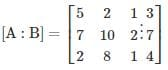by applying row transformation R→ R− (R1+R3), we get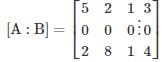so, rank of matrix = rank of augmented matrix = 2 < number of variables

∴ System has infinite solutions

Alternatively we can simply observe that second equation is redundant as it can be obtained from rest two. Hence we have 2 primary equations and 3 variables hence infinite solution.

QUESTION: 3

### Matrix for which LU decomposition is not possible?

Solution:

An invertible matrix A has an LU decomposition provided that all it's leading submatrices have non-zero determinants.

All the matrices in the options are invertible because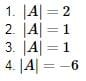In option 4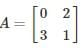in this leading sub matrix A = 
Hence LU decomposition is not possible

*Answer can only contain numeric values
QUESTION: 4

If the coefficient matrix is Cn×n and its corresponding rank is c also the augmented matrix is An×(n+1) and its corresponding rank is a, then how many statements given below are incorrect?

I. If c ≠ a, the equations are inconsistent with an infinite number of solutions.

II. If c = a < n, the equations are inconsistent

III. If c = a =n, equation may be inconsistent.

Solution:
• If c ≠ a, the equations are inconsistent i.e.  no solution possible
• If c = a < n, the equations are consistent with infinite number of solutions
• If c = a =n, the equations are consistent and there is a unique solution.
QUESTION: 5

Find the value of x and y for the below given simultaneous equations that has no solutions?

a + b + c = 18

2a + 4b + 6c = 12

2a + xc + 4b = y

Solution:

For given system of equations to have no solution, the determinant of the coefficient matrix should be equal to 0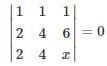1 × (4x−24) − 1 × (2x−12) + 1(8−8) = 0

2x − 12 = 0

∴ x = 6
Augmented matrix is given by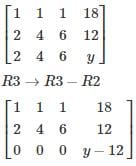For given system of equations to have no solution, rank of augmented matrix should be greater than coefficient matrix
∴ y − 12 ≠ 0
∴ y ≠ 12

*Answer can only contain numeric values
QUESTION: 6

In the LU decomposition of the matrix,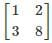, if the diagonal elements of U are both 1, then the trace of L is:

Solution: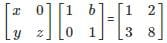We get x = 1 and z = 2.

Hence tr(L) = 3.

QUESTION: 7

Consider the following system of equations in three real variables x, y, z.

2x – 3y + 7z = 5

3x + y – 3z = 13

2x + 19y – 47z = 32

The system of the equation has

Solution:

Augmented matrix will be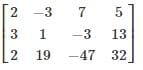R3 → R3 – R1

R2 → 2R2 – 3R1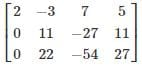R3 → R3 – 2R2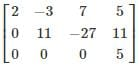Rank of A ≠ Rank of Augmented matrix

Hence given system of equations has no solution.

QUESTION: 8

Among the given two sets which is/are consistent:
S1 = {3x + ay + 4z = 0,bx + 2y + z = 0,5x + 7z + 9z = 0
S2 = {2x + 6y= −11, 6x + 20y − 6z = −3, 6y − 18z = −1}

Solution:

Set S1 contains homogeneous equations, homogeneous equations are always consistent because it always satisfied a trivial solution i.e. x = y = z = 0

For S2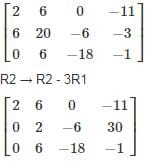R2 → 3R2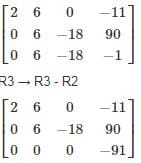Rank Augmented matrix is 3 while rank of coefficient matrix has rank is 2

Hence the S2 is inconsistent

∴ only S1 is consistent

QUESTION: 9

The number of values of k for which the system of equations (k + 1) x + 8y = 4k, kx + (k + 3)y = 3k – 1 has infinitely many solutions, is

Solution:

(k + 1)x + 8y = 4k

kx + (k + 3)y = 3k – 1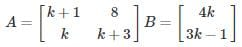The given system of linear equations has infinitely many solutions if ρ(A) = ρ(A|B) < n = 2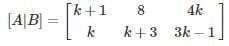To get the rank less than 2, one row should be dependent on another.

nk = (k + 1), n(k + 3) = 8, n(3k – 1) = 4k

by solving the above equations, we get

n = 2, k = 1

Hence for k = 1 only the system has infinitely many solutions.

QUESTION: 10

The simultaneous equations

2x + ay + z = 20

x + 3y + 4z = b

x + 2y + 3z = c

has unique solution then what is the value of a, b and c respectively?

Solution:

For given system of equations to have unique solution, the determinant of the coefficient matrix should be not equal to 0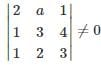2 (9−8) − a(3−4) + (2−3) ≠ 0

2 + a − 1 ≠ 0

a ≠ −1

Since Δ of coefficient matrix is not equal to 0 then b and c can take any value

Note:

If coefficient matrix’s Δ ≠ 0 then rank of coefficient matrix is always equal rank of augmented matrix so right-hand side of given equation can take any valueUse Code STAYHOME200 and get INR 200 additional OFF Use Coupon Code